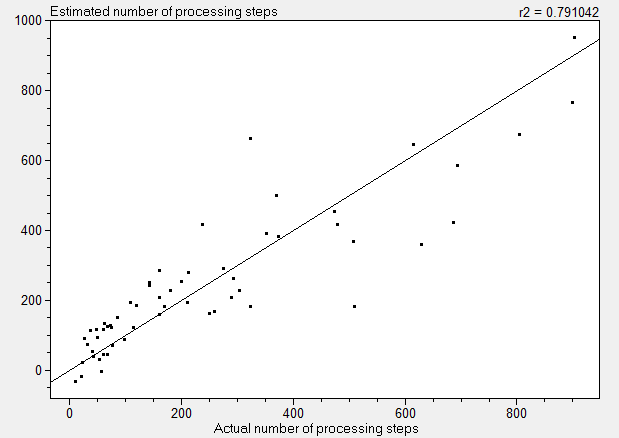The SDL Component Suite is an industry leading collection of components supporting scientific and engineering computing. Please visit the SDL Web site for more information....## kMeansEstimatedSteps

 Unit: SDL_math2 Class: none Declaration: function kMeansEstimatedSteps (NumObjects, NumClusters, NumVars: integer): integer;

The maximum number of processing steps required to perform a kMeans cluster analysis (reflected by the global variable ProcStat) depends on several factors and cannot be specified exactly. The function kMeansEstimatedSteps returns an estimate of the maximum number of processing steps which can be used, for example, to scale a progress bar. The parameters NumObjects, NumClusters, and NumVars specify the number of objects, the number of clusters and the number of involved variables, respectively.

Please note that due to the high variability in the model data (which in turn depend on the structure of the dataset to be clustered) the estimation is based on a regression model which exhibits a rather low quality of fit (r2 approx. 0.79). Thus the estimated number of processing steps is more or less a guess which may be wrong by more than 50%. If the estimated value is less than 10 it is automatically restricted to 10. The following diagram shows the plot of estimated values against actual values for the dataset used to set up the regression model:Last Update: 2015-Mar-26